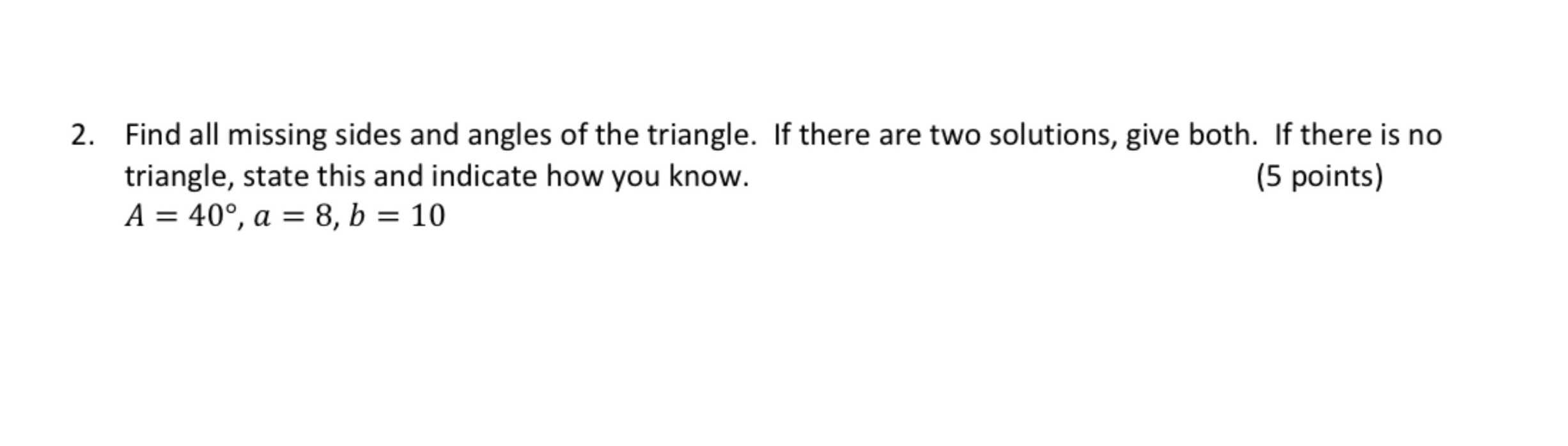### Still have math questions?

Trigonometry
QuestionFind all missing side and angle of the triangle. If there are two solutions,given both if there is no triangle,state this and indicate how you know A=40 °, a=8, b=10

2. Find all missing sides and angles of the triangle. If there are two solutions, give both. If there is no triangle, state this and indicate how you know.

$$A = 40 ^ { \circ } , a = 8 , b = 10$$

(5 points)

Acute Scalene Triangle Side $$a = 8$$ Side $$b = 10$$

Side $$c = 12.42305$$

Angle $$\angle A = 40 ^ { \circ } = 0.69813 rad = 2 / 9 \pi$$

Angle $$\angle B = 53.464 ^ { \circ } = 53 ^ { \circ } 27 ^ { \prime } 51 ^ { \prime \prime } = 0.93313 rad$$

Angle $$\angle C = 86.536 ^ { \circ } = 86 ^ { \circ } 32 ^ { \prime } 9 ^ { \prime \prime } = 1.51034 rad$$

Obtuse Scalene Triangle Side $$a = 8$$ Side $$b = 10$$

Side $$c = 2.89784$$

Angle $$\angle A = 40 ^ { \circ } = 0.69813 rad = 2 / 9 \pi$$

Angle $$\angle B = 126.536 ^ { \circ } = 126 ^ { \circ } 32 ^ { \prime } 9 ^ { \prime \prime } = 2.20847 rad$$

Angle $$\angle C = 13.464 ^ { \circ } = 13 ^ { \circ } 27 ^ { \prime } 51 ^ { \prime \prime } = 0.23499 rad$$

Solution
View full explanation on CameraMath App.